• +91 9971497814
• info@interviewmaterial.com

### Related Subjects

Question 1 :

The angles ofquadrilateral are in the ratio 3 : 5 : 9 : 13. Find all the angles of thequadrilateral.

Let the common ratio between the angles be = x.

We know that the sum of the interior angles of the quadrilateral= 360°

Now,

3x+5x+9x+13x = 360°

30x =360°

x =12°

, Angles of the quadrilateral are:

3x = 3×12° = 36°

5x = 5×12° = 60°

9x = 9×12° = 108°

13x = 13×12° = 156°

Question 2 :

If the diagonals ofa parallelogram are equal, then show that it is a rectangle.

Answer 2 :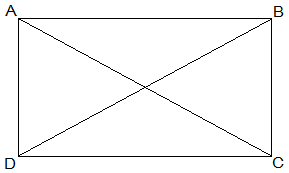Given that,

AC = BD

To show that, ABCD is a rectangle if the diagonals of aparallelogram are equal

To show ABCD is a rectangle we have to prove that one of itsinterior angles is right angled.

Proof,

BC = BA (Common)

AC = AD (Opposite sides of a parallelogram are equal)

AC = BD (Given)

A = B [Corresponding parts of CongruentTriangles]

also,

A+B = 180° (Sum of the angles on the sameside of the transversal)

2A = 180°

A = 90° = B

, ABCD is a rectangle.

Hence Proved.

Question 3 : Show that if the diagonals of a quadrilateral bisecteach other at right angles, then it is a rhombus.

Answer 3 :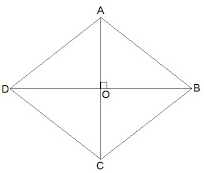Let ABCD be a quadrilateral whose diagonals bisect each other atright angles.

Given that,

OA = OC

OB = OD

and AOB = BOC = OCD = ODA =90°

To show that,

if the diagonals of a quadrilateral bisect each other at rightangles, then it is a rhombus.

i.e., we have to prove that ABCD is parallelogram and AB = BC =CD = AD

Proof,

In ΔAOB and ΔCOB,

OA = OC (Given)

AOB = COB (Opposite sides of a parallelogram areequal)

OB = OB (Common)

Therefore, ΔAOB ΔCOB[SAS congruency]

Thus, AB = BC [CPCT]

Similarly we can prove,

BC = CD

, AB = BC = CD = AD

Opposites sides of a quadrilateral are equal hence ABCD is aparallelogram.

, ABCD is rhombus as it is a parallelogram whose diagonalsintersect at right angle.

HenceProved.

Question 4 :

Show that thediagonals of a square are equal and bisect each other at right angles.

Answer 4 :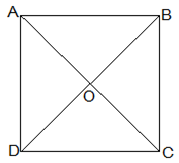Let ABCD be a square and its diagonals AC and BD intersect eachother at O.

To show that,

AC = BD

AO = OC

and AOB =90°

Proof,

AB = BA (Common)

Thus,

AC = BD [CPCT]

diagonals are equal.

Now,

In ΔAOB and ΔCOD,

BAO = DCO (Alternate interior angles)

AOB = COD (Vertically opposite)

AB = CD (Given)

, ΔAOB ΔCOD[AAS congruency]

Thus,

AO = CO [CPCT].

, Diagonal bisect each other.

Now,

In ΔAOB and ΔCOB,

OB = OB (Given)

AO = CO (diagonals are bisected)

AB = CB (Sides of the square)

, ΔAOB ΔCOB[SSS congruency]

also, AOB = COB

AOB+COB = 180° (Linear pair)

Thus, AOB = COB = 90°

,Diagonals bisect each other at right angles

Question 5 :

Show that if thediagonals of a quadrilateral are equal and bisect each other at right angles,then it is a square.

Answer 5 :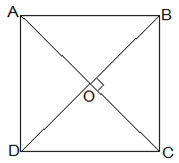Given that,

Let ABCD be a quadrilateral and its diagonals AC and BD bisecteach other at right angle at O.

To prove that,

The Quadrilateral ABCD is a square.

Proof,

In ΔAOB and ΔCOD,

AO = CO (Diagonals bisect each other)

AOB = COD (Vertically opposite)

OB = OD (Diagonals bisect each other)

, ΔAOB ΔCOD[SAS congruency]

Thus,

AB = CD [CPCT] — (i)

also,

OAB = OCD (Alternate interior angles)

AB ||CD

Now,

In ΔAOD and ΔCOD,

AO = CO (Diagonals bisect each other)

AOD = COD (Vertically opposite)

OD = OD (Common)

, ΔAOD ΔCOD[SAS congruency]

Thus,

AD = CD [CPCT] — (ii)

also,

AD =BC = CD = AB — (ii)

and ADC+BCD = 180° (co-interior angles)

One of the interior angles is right angle.

Thus, from (i), (ii) and (iii) given quadrilateral ABCD is asquare.

Hence Proved.

Question 6 :

Diagonal AC of aparallelogram ABCD bisects A (see Fig. 8.19). Show that

(i) it bisects C also,

(ii) ABCD is a rhombus.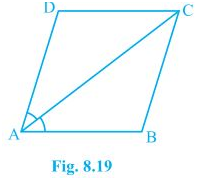AD = CB (Opposite sides of a parallelogram)

DC = BA (Opposite sides of a parallelogram)

AC = CA (Common Side)

Thus,

ACD = CAB by CPCT

ACD = BCA

Thus,

AC bisects C also.

(ii) ACD = CAD (Proved above)

AD =CD (Opposite sides of equal angles of a triangle are equal)

Also, AB = BC = CD = DA (Opposite sides of a parallelogram)

Thus,

ABCDis a rhombus.

Question 7 :

ABCD is arhombus. Show that diagonal AC bisects A as well as C and diagonal BD bisects B as well as D.

Answer 7 :Given that,

ABCD is a rhombus.

AC and BD are its diagonals.

Proof,

AD = CD (Sides of a rhombus)

DAC = DCA (Angles opposite of equal sides of atriangle are equal.)

also, AB || CD

⇒∠DAC = BCA (Alternate interior angles)

⇒∠DCA = BCA

, AC bisects C.

Similarly,

We can prove that diagonal AC bisects A.

Following the same method,

Wecan prove that the diagonal BD bisects Band D.

Question 8 :

ABCD is arectangle in which diagonal AC bisects A as well as C. Show that:

(i) ABCD is a square

(ii) Diagonal BD bisects B as well as D.

Answer 8 :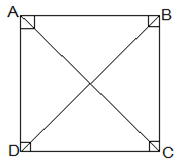(i) DAC = DCA (AC bisects A as well as C)

AD =CD (Sides opposite to equal angles of a triangle are equal)

also, CD = AB (Opposite sides of a rectangle)

,AB = BC = CD = AD

Thus, ABCD is a square.

(ii) In ΔBCD,

BC = CD

CDB = CBD (Angles opposite to equal sides are equal)

also, CDB = ABD (Alternate interior angles)

CBD = ABD

Thus, BD bisects B

Now,

Thus,BD bisects D

Question 9 :

In parallelogramABCD, two points P and Q are taken on diagonal BD such that DP = BQ (see Fig.8.20). Show that:

(i) ΔAPD ΔCQB

(ii) AP = CQ

(iii) ΔAQB ΔCPD

(iv) AQ = CP

(v) APCQ is a parallelogram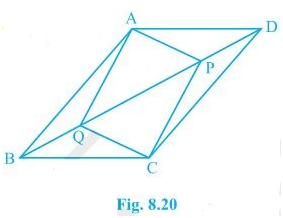(i) In ΔAPD and ΔCQB,

DP = BQ (Given)

ADP = CBQ (Alternate interior angles)

AD = BC (Opposite sides of a parallelogram)

Thus, ΔAPD ΔCQB[SAS congruency]

(ii) AP = CQ by CPCT as ΔAPD ΔCQB.

(iii) In ΔAQB and ΔCPD,

BQ = DP (Given)

ABQ = CDP (Alternate interior angles)

AB = CD (Opposite sides of a parallelogram)

Thus, ΔAQB ΔCPD[SAS congruency]

(iv) As ΔAQB ΔCPD

AQ = CP [CPCT]

(v) From the questions (ii) and (iv), it is clear that APCQ hasequal opposite sides and also has equal and opposite angles. , APCQ is aparallelogram.

Question 10 :

ABCD is aparallelogram and AP and CQ are perpendiculars from vertices A and C ondiagonal BD (see Fig. 8.21). Show that

(i) ΔAPB ΔCQD

(ii) AP = CQ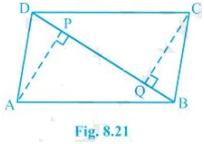(i) In ΔAPB and ΔCQD,

ABP = CDQ (Alternate interior angles)

APB = CQD (= 90o asAP and CQ are perpendiculars)

AB = CD (ABCD is a parallelogram)

, ΔAPB ΔCQD[AAS congruency]

(ii) As ΔAPB ΔCQD.

,AP = CQ [CPCT]

Todays Deals### Chapter 8 Quadrilaterals Ex-8.1 Contributorskrishan

Name:
Email:

# Latest News# 9000 interview questions in different categories